# 如何用Qt绘制一颗好看的二叉树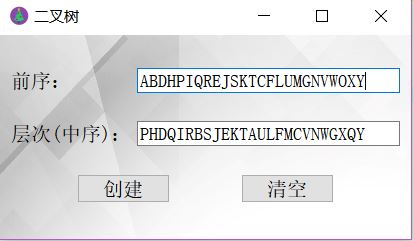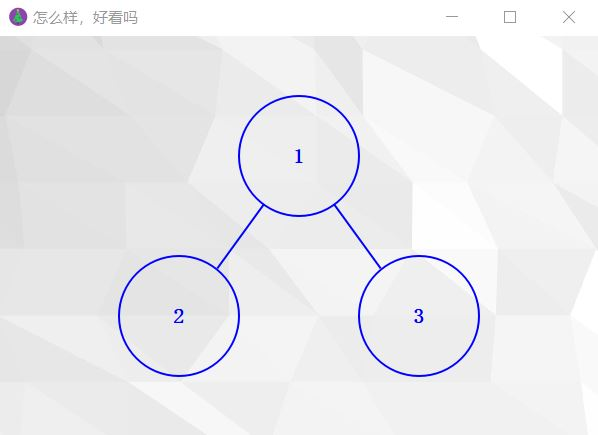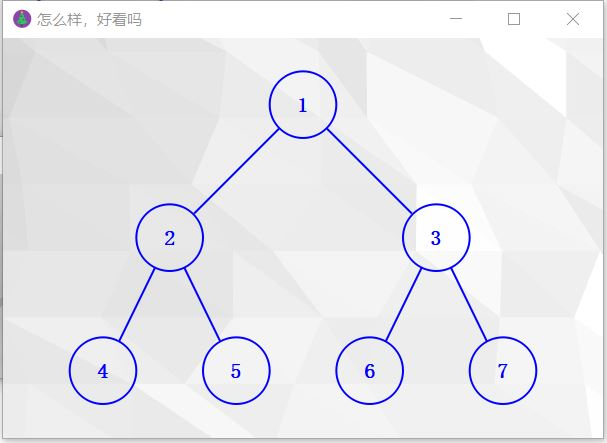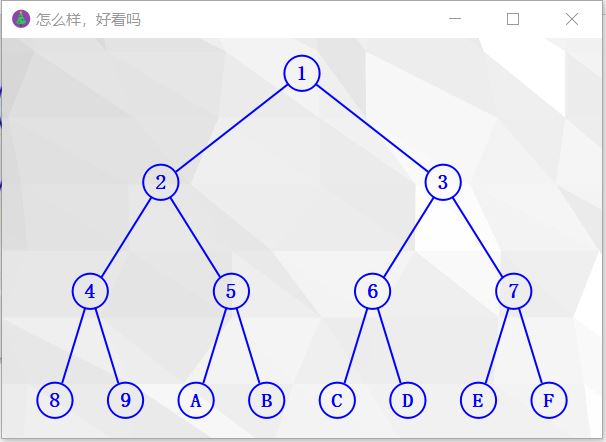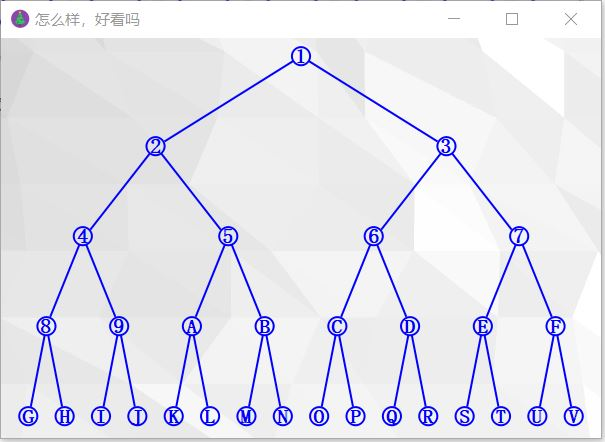OK，让我们开始吧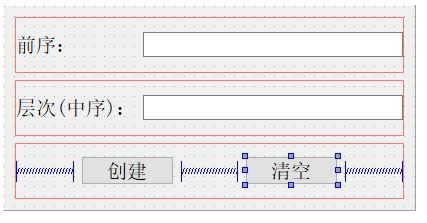void Widget::on_btnCreat_clicked()
{
QString input1 = ui->lEdInput1->text();
QString input2 = ui->lEdInput2->text();

myPaint *p = new myPaint;
if (p->setInput(input1, input2))
p->show();
else
QMessageBox::warning(this, "警告", "无效的输入");
}

myPaint类拥有公有成员函数setInput()，保护成员函数paintEvent()，以及私有成员myTree，代码如下

public:
explicit myPaint(QWidget *parent = 0);
bool setInput(QString input1, QString input2);

protected:
void paintEvent(QPaintEvent *);

private:
linkedBinaryTree<char>* myTree;

myTree的数据类型就是你自定义的二叉树。

myPaint::myPaint(QWidget *parent) : QWidget(parent)
{
resize(600, 400);
setWindowTitle("怎么样，好看吗");
setWindowIcon(QIcon("://image/tree.png"));

}

bool myPaint::setInput(QString input1, QString input2)
{
// 将QString转化为C++标准模板库里的String
std::string s1 = input1.toStdString();
std::string s2 = input2.toStdString();
try {
if (s1 != "" && s2 != "")
{
myTree->preAndInCreatBinaryTree(s1, s2);
}
else if (s1 != "")
{
myTree->preCreatBinaryTree(s1);
}
else if (s2 != "")
{
myTree->levelCreatBinaryTree(s2);
}
}
catch (invalidSequence) {
return false;
}
return true;
}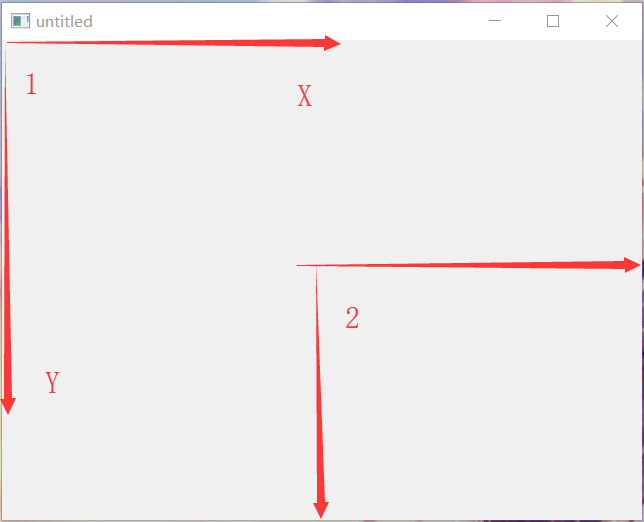translate()函数提供了坐标系统平移的功能。假使我们用W表示当前窗口的宽度，用H表示当前窗口的高度，那么

translate(W/2, H/2)就可以将坐标系统的原点平移至上图的位置2。这时（0, 0）坐标点就表示窗口的中点，（-W/2, -H/2）就表示窗口的左上点，是不是很好理解？

rotate()函数可以旋转坐标系统。如rotate(45)可以将坐标系统顺时针旋转45度；rotate(-45)可以将坐标系统逆时针旋转45度。

OK，接下来我们分析一下一颗二叉树在窗口中的位置，如下图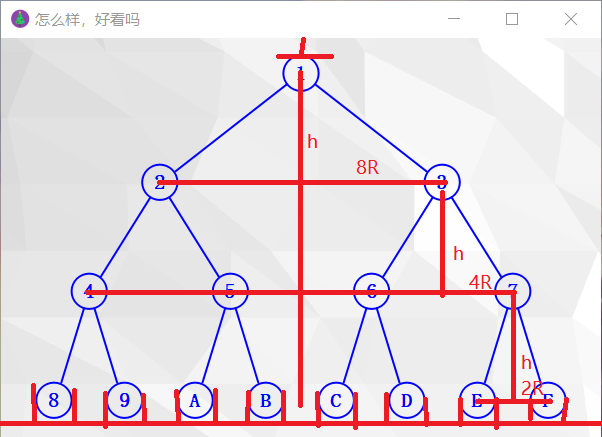W = (8 + 9) * 2 * R，很好理解吧。而8个节点又和二叉树的高度有关，如果我们用treeHeight表示二叉树的高度，则

W = (2 ^ (treeHeight - 1) + (2 ^ (treeHeight - 1) + 1)) * 2 * R。蓝色部分是节点个数8，红色部分是空位9

qreal W = this->width();                            // 画布的宽
qreal H = this->height();                           // 画布的高
int treeHeight = myTree->getHeight();               // 树的高度
qreal R = W / (2 * std::pow(2, treeHeight) + 2);    // 节点的半径

const int layerHeight = (H-4*R) / (treeHeight-1);     // 层高，即垂直偏移量

struct stackNode
{
binaryTreeNode<char>* treeNode;
int layer;      // 标记该节点属于第几层
};

// 初始化
// 节点的定义
QRectF node(QPointF(-R, -R), QPointF(R, R));
arrayStack<stackNode> stack;    // 存放右孩子节点
stackNode qNode;

arrayStack<QPointF> points;     // 存放右孩子节点相对于当前坐标系统原点的位置
QPointF point;

qreal Hoffset;                  // 水平偏移量
binaryTreeNode<char>* t = myTree->getRoot();
const qreal Pi = 3.14159;
int curLayer = 1;
int curAngle;                   // 当前角度
qreal deg;                      // 当前弧度

// 将坐标系统的原点（下文简称原点）移动到初始位置
painter.translate(W/2, 2*R);

while (1)
{
painter.drawEllipse(node);
painter.drawText(node, Qt::AlignCenter, QString(t->element));

// 设置孩子节点相对于父节点的水平偏移量
Hoffset = std::pow(2, (treeHeight - curLayer)) * R;
deg = std::atan(Hoffset / layerHeight);             // 返回的是弧度
curAngle = 180 / Pi * deg;                          // 将弧度转化为角度

if (t->rightChild != NULL)
{
// 坐标轴逆时针旋转
painter.rotate(-curAngle);

//绘制图形路径
painter.drawLine(0, R, 0, layerHeight / std::cos(deg) - R);

// 旋转复原
painter.rotate(curAngle);

// 右孩子节点压栈
qNode.treeNode = t->rightChild;
qNode.layer = curLayer + 1;
stack.push(qNode);

// 右孩子相对于当前坐标系统原点的位置压栈
points.push(QPointF(QPointF(0, 0) + QPointF(Hoffset, layerHeight)));
painter.save();
}
if (t->leftChild != NULL)
{
// 坐标轴顺时针旋转
painter.rotate(curAngle);
// 绘制边
painter.drawLine(0, R, 0, layerHeight / std::cos(deg) - R);
// 旋转复原
painter.rotate(-curAngle);
// 原点移动到左孩子节点的位置
painter.translate(QPointF(QPointF(0, 0) + QPointF(-Hoffset, layerHeight)));
t = t->leftChild;
// 层次加1
curLayer++;
}
else {
try {
// 获取到右节点的层次状态
stack.pop(qNode);
t = qNode.treeNode;
curLayer = qNode.layer;

// 原点移动到右孩子节点的位置
painter.restore();
points.pop(point);
painter.translate(point);
}
catch (stackEmpty) { painter.resetTransform(); return; }
}
}

Hoffset = 2 ^ (treeHeight - curLayer) * R

painter.drawLine(0, R, 0, layerHeight / std::cos(deg) - R);

#include "mypaint.h"
#include <QPainter>
#include <QIcon>

struct stackNode
{
binaryTreeNode<char>* treeNode;
int layer;      // 标记该节点属于第几层
};

myPaint::myPaint(QWidget *parent) : QWidget(parent)
{
resize(600, 400);
setWindowTitle("怎么样，好看吗");
setWindowIcon(QIcon("://image/tree.png"));

}

bool myPaint::setInput(QString input1, QString input2)
{
// 将QString转化为C++标准模板库里的String
std::string s1 = input1.toStdString();
std::string s2 = input2.toStdString();
try {
if (s1 != "" && s2 != "")
{
myTree->preAndInCreatBinaryTree(s1, s2);
}
else if (s1 != "")
{
myTree->preCreatBinaryTree(s1);
}
else if (s2 != "")
{
myTree->levelCreatBinaryTree(s2);
}
}
catch (invalidSequence) {
return false;
}
return true;
}

void myPaint::paintEvent(QPaintEvent *)
{
//创建QPainter对象
QPainter painter(this);

// 反锯齿
painter.setRenderHint(QPainter::Antialiasing);
painter.setRenderHint(QPainter::TextAntialiasing);

//背景图
painter.drawPixmap(rect(), QPixmap("://image/600_400.jpg"));

//设置字体
QFont font;
font.setPointSize(12);
font.setBold(true);
painter.setFont(font);

//设置画笔
QPen penLine;
penLine.setWidth(2); //线宽
penLine.setColor(Qt::blue); //划线颜色
penLine.setStyle(Qt::SolidLine);//线的类型，实线、虚线等
penLine.setCapStyle(Qt::FlatCap);//线端点样式
penLine.setJoinStyle(Qt::BevelJoin);//线的连接点样式
painter.setPen(penLine);

qreal W = this->width();                            // 画布的宽
qreal H = this->height();                           // 画布的高
int treeHeight = myTree->getHeight();               // 树的高度
qreal R = W / (2 * std::pow(2, treeHeight) + 2);    // 节点的半径

const int layerHeight = (H-4*R) / (treeHeight-1);     // 层高，即垂直偏移量

// 初始化
// 节点的定义
QRectF node(QPointF(-R, -R), QPointF(R, R));
arrayStack<stackNode> stack;    // 存放右孩子节点
stackNode qNode;

arrayStack<QPointF> points;     // 存放右孩子节点相对于当前坐标系统原点的位置
QPointF point;

qreal Hoffset;                  // 水平偏移量
binaryTreeNode<char>* t = myTree->getRoot();
const qreal Pi = 3.14159;
int curLayer = 1;
int curAngle;                   // 当前角度
qreal deg;                      // 当前弧度

// 将坐标系统的原点（下文简称原点）移动到初始位置
painter.translate(W/2, 2*R);

while (1)
{
painter.drawEllipse(node);
painter.drawText(node, Qt::AlignCenter, QString(t->element));

// 设置孩子节点相对于父节点的水平偏移量
Hoffset = std::pow(2, (treeHeight - curLayer)) * R;
deg = std::atan(Hoffset / layerHeight);             // 返回的是弧度
curAngle = 180 / Pi * deg;                          // 将弧度转化为角度

if (t->rightChild != NULL)
{
// 坐标轴逆时针旋转
painter.rotate(-curAngle);

//绘制图形路径
painter.drawLine(0, R, 0, layerHeight / std::cos(deg) - R);

// 旋转复原
painter.rotate(curAngle);

// 右孩子节点压栈
qNode.treeNode = t->rightChild;
qNode.layer = curLayer + 1;
stack.push(qNode);

// 右孩子相对于当前坐标系统原点的位置压栈
points.push(QPointF(QPointF(0, 0) + QPointF(Hoffset, layerHeight)));
painter.save();
}
if (t->leftChild != NULL)
{
// 坐标轴顺时针旋转
painter.rotate(curAngle);
// 绘制边
painter.drawLine(0, R, 0, layerHeight / std::cos(deg) - R);
// 旋转复原
painter.rotate(-curAngle);
// 原点移动到左孩子节点的位置
painter.translate(QPointF(QPointF(0, 0) + QPointF(-Hoffset, layerHeight)));
t = t->leftChild;
// 层次加1
curLayer++;
}
else {
try {
// 获取到右节点的层次状态
stack.pop(qNode);
t = qNode.treeNode;
curLayer = qNode.layer;

// 原点移动到右孩子节点的位置
painter.restore();
points.pop(point);
painter.translate(point);
}
catch (stackEmpty) { painter.resetTransform(); return; }
}
}
}

09-13
02-0706-06115
12-29
03-021282
05-282647
10-152180
11-12